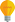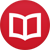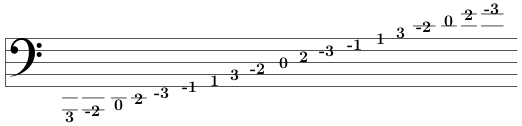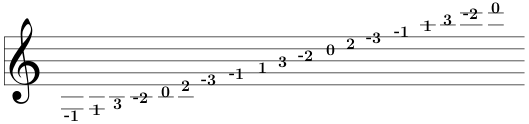# Meaning of accidentals and key signatures in terms of tonal distance

by Jakub MarianTip: See my list of theMost Common Mistakes in English. It will teach you how to avoid mis­takes with com­mas, pre­pos­i­tions, ir­reg­u­lar verbs, and much more.

In the previous article, we learned how we can translate note names into numbers using the following table:

 … D♭ A♭ E♭ B♭ F C G D A E B F♯ C♯ G♯ D♯ … … -7 -6 -5 -4 -3 -2 -1 0 1 2 3 4 5 6 7 …

But what do the sharps and flats mean? If we look at the numbers, the relation becomes apparentfor example, F = $-3$, F♯ = $4$; D = $0$, D♯ = $7$, so:

The ♯ sign means +7 in the numeral notation.

Of course, the same works for the flat sign too:

The ♭ sign means –7 in the numeral notation.

This explains how the traditional staff notation with key signatures works. A plain staff contains only notes from $-3$ to $3$, i.e. the C major/A minor segment of the table above. Other scales require “shifting” of this range; for example, D major requires the notes from $-1$ to $5$. The notes $-3$ and $-2$ are no longer required, but the notes $4$ and $5$ are missing; by applying the ♯ sign to $-3$ and $-2$, we obtain $-3$♯ $= -3+7 = 4$ and $-2$♯ $= -2+7 = 5$, which were the “missing” notes. We have therefore a simple rule of how to translate numerals into the staff notation:

In the staff notation, the notes $4$, $5$, $6$, … would be written as $-3$♯, $-2$♯, $-1$♯, … . The notes $-4$, $-5$, $-6$, …, would be written as $3$♭, $2$♭, $1$♭, … .

Of course, one has to learn where the numerals representing notes are located on the staff to be able to read it. It is perhaps easier to learn the bass clef first, because the numbers are “symmetric” around the middle line, and the first line and space from both top and bottom are either 1 or -1:The treble clef can be remembered as the bass clef shifted “one line up”:Once we remember the numeral representation of notes on the staff, writing any key signature becomes trivial. For example $3$ major (B major) has $3+2=5$ sharps, so we would write the ♯ sign to $-3$, $-2$, $-1$, $0$, and $1$, and this is actually the exact order in which the accidentals are traditionally written:We have seen that writing key signatures becomes trivial, but what about reading them? If you read the previous article, you have seen that this is actually even more trivialone just has to count the accidentals (counting flats as negative):

The key signature with $n$ accidentals represents either the $n-2$ major scale or the $n+1$ minor scale.
So, for example, if you see 5 sharps and know that the scale is major, you immediately know that it is $5-2 = 3$ major.

By the way, I have written several educational ebooks. If you get a copy, you can learn new things and support this website at the same time—why don’t you check them out?